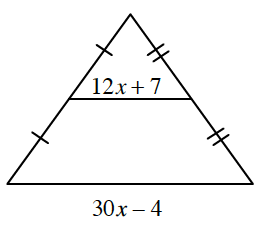Home > CCG > Chapter 9 > Lesson 9.1.5 > Problem9-59

9-59.

Write and solve an equation based on the geometric relationship shown below. Homework Help ✎Although you do not have dimensions, you can write an equation by examining the relationship between the side lengths.

$2(12x + 7) = 30x − 4$, so $x = 3$.